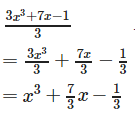Courses

# Test: Relations & Functions - 1

## 25 Questions MCQ Test Mathematics (Maths) Class 12 | Test: Relations & Functions - 1

Description
This mock test of Test: Relations & Functions - 1 for JEE helps you for every JEE entrance exam. This contains 25 Multiple Choice Questions for JEE Test: Relations & Functions - 1 (mcq) to study with solutions a complete question bank. The solved questions answers in this Test: Relations & Functions - 1 quiz give you a good mix of easy questions and tough questions. JEE students definitely take this Test: Relations & Functions - 1 exercise for a better result in the exam. You can find other Test: Relations & Functions - 1 extra questions, long questions & short questions for JEE on EduRev as well by searching above.
QUESTION: 1

### If R is a relation from a non – empty set A to a non – empty set B, then

Solution:

Let A and B be two sets. Then a relation R from set A to set B is a subset of A × B. Thus, R is a relation from A to B ⇔ R ⊆ A × B.

QUESTION: 2

### The range of the function f(x) = 7-x Px-3 is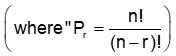Solution:

Here, 0 ≤ x- 3 ≤ 7 - x
⇒0 ≤ x - 3 and x - 3 ≤ 7 - x
By solvation, we will get 3 ≤ x ≤ 5
So x = 3,4,5 find the values of 7-x Px - 3 by substituting the values of x

QUESTION: 3

### Let R be the relation over the set of straight lines of a plane such that l1 R l2 ⇔ l1 ⊥ l2. Then, R is

Solution:

To be reflexive, a line must be perpendicular to itself, but which is not true. So, R is not reflexive
For symmetric, if  l1 R l2 ⇒ l1 ⊥ l2.
⇒  l2 ⊥ l1 ⇒ l1 R l2 hence symmetric
For transitive,  if l1 R l2 and l2 R l3
⇒ l1 R l2  and l2 R l3  does not imply that l1 ⊥ l3 hence not transitive.

QUESTION: 4

The diagram given below shows that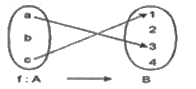Solution:

Because, the element b in the domain A has no image in the co-domain B.

QUESTION: 5

Which of the following is an even function?

Solution:

Because, f(- x) = f(x) is the necessary condition for a function to be an even function, which is only satisfied by x2+ sin2x .

QUESTION: 6

The binary relation S = Φ (empty set) on set A = {1, 2, 3} is

Solution:

Reflexive : A relation is reflexive if every element of set is paired with itself. Here none of the element of A is paired with themselves, so S is not reflexive.
Symmetric : This property says that if there is a pair (a, b) in S, then there must be a pair (b, a) in S. Since there is no pair here in S, this is trivially true, so S is symmetric.
Transitive : This says that if there are pairs (a, b) and (b, c) in S, then there must be pair (a,c) in S. Again, this condition is trivially true, so S is transitive.

QUESTION: 7

The void relation (a subset of A x A) on a non empty set A is:

Solution:

The relation { } ⊂ A x A on a is surely not reflexive. However, neither symmetry nor transitivity is contradicted. So { } is a transitive and symmetry relation on A.

QUESTION: 8

A relation R in a set A is called reflexive,

Solution:

A relation R on a non empty set A is said to be reflexive if fx Rx for all x ∈ R, Therefore, R is reflexive.

QUESTION: 9

The domain of the function f = {(1, 3), (3, 5), (2, 6)} is

Solution:

The domain in ordered pair (x,y) is represented by x-coordinate. Therefore, the domain of the given function is given by : {1, 3, 2}.

QUESTION: 10

The domain of the function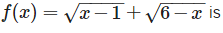Solution:

x - 1 ≥ 0 and 6 – x ≥ 0 ⇒ 1 ≤ x ≤ 6.

QUESTION: 11

Let R be the relation on N defined as x R y if x + 2 y = 8. The domain of R is

Solution:

As x R y if x + 2y = 8, therefore, domain of the relation R is given by x = 8 – 2y ∈ N.
When y = 1,
⇒ x = 6 ,when y = 2,
⇒ x = 4, when y = 3,
⇒ x = 2.
therefore domain is {2, 4, 6}.

QUESTION: 12

If n ≥ 2, then the number of onto mappings or surjections that can be defined from {1, 2, 3, 4, ……….., n} onto {1, 2} is

Solution:

The number of onto functions that can be defined from a finite set A containing n elements onto a finite set B containing 2 elements = 2− 2.

QUESTION: 13

A relation R in a set A is called symmetric, if

Solution:

A relation R on a non empty set A is said to be symmetric if fx Ry ⇔ yRx, for all x , y ∈ R .

QUESTION: 14

The range of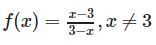is

Solution:

We have ,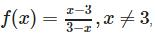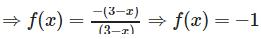Therefore, range of f(x) is {-1}.

QUESTION: 15

The function f(x) = sin x2 is

Solution:

For even function: f(-x) = f(x) ,
therefore, f(− x)
= sin (− x)2 = sin x2 = f(x).

QUESTION: 16

Which of the following is not an equivalence relation on I, the set of integers ; x, y

Solution:

If R is a relation defined by xRy : ifx ⩽ y, then R is reflexive and transitive But, it is not symmetric. Hence, R is not an equivalence relation.

QUESTION: 17

If A = {1, 2, 3}, then the relation R = {(1, 2), (2, 3), (1, 3) in A is

Solution:

A relation R on a non empty set A is said to be transitive if fxRy and y Rz ⇒ xRz, for all x ∈ R. Here, (1, 2) and (2, 3) belongs to R implies that (1, 3) belongs to R.

QUESTION: 18

A relation R in a set A is called transitive, if

Solution:

A relation R on a non empty set A is said to be transitive if fx Ry and yRz ⇒ x Rz, for all x ∈ R.

QUESTION: 19

The range of the function f(x) =|x−1| is

Solution:

We have, f(x) = |x−1|, which always gives non-negative values of f(x) for all x ∈ R.Therefore range of the given function is all non-negative real numbers i.e. [0,∞).

QUESTION: 20

The range of the function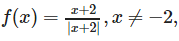is

Solution:

As the denominator of the functionis a modulus function i.e.

QUESTION: 21

Let A = {a, b, c} and R = {(a, a), (b, b), (c, c), (b, c)} be a relation on A. Here, R is

Solution:

Correct Answer :- b

Explanation:- A = {a, b, c} and R = {(a, a), (b, b), (c, c), (b, c)}

Any relation R is reflexive if fx Rx for all x ∈ R. Here ,(a, a), (b, b), (c, c) ∈ R. Therefore , R is reflexive.

For the transitive, in the relation R there should be (a,c)

Hence it is not transitive.

QUESTION: 22

A relation R from C to R is defined by x Ry iff |x| = y. Which of the following is correct?

Solution: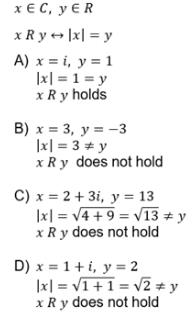QUESTION: 23

A relation R in a set A is said to be an equivalence relation if

Solution:

A relation R on a non empty set A is said to be reflexive iff xRx for all x ∈ R . .
A relation R on a non empty set A is said to be symmetric if fx Ry ⇔ y Rx, for all x , y ∈ R .
A relation R on a non empty set A is said to be transitive if fx Ry and y Rz ⇒ x Rz, for all x ∈ R.
An equivalence relation satisfies all these three properties.

QUESTION: 24

Let f: R → R be a mapping such that f(x) =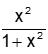. Then f is

Solution:
QUESTION: 25

Which of the following is a polynomial function?

Solution:

A polynomial function has all exponents as integral whole numbers.